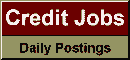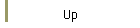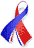DefaultRisk.com the web's biggest credit risk modeling resource.doi> search: A or B Export citation to:- HTML- Text (plain)- BibTeX- RIS- ReDIF

Loss Distribution Evaluation for Synthetic CDOs

by Ken Jackson of the University of Toronto,
Alex Kreinin of Algorithmics, Inc., and
Xiaofang Ma of the University of Toronto

February 12, 2007

Abstract: Efficient numerical methods for evaluating the loss distributions of synthetic CDOs are important for both pricing and risk management purposes. In this paper we discuss several methods for loss distribution evaluations. We first develop a stable recursive method. Then the improved compound Poisson approximations proposed by Hipp are introduced. Finally, the normal power approximation method that has been used in actuarial science is described. The recursive method computes the loss distribution exactly, whereas the other two methods compute the loss distribution approximately. Numerical results based on these three and some known methods for synthetic CDO pricing are given.

Keywords: synthetic CDO, loss distribution, recursive method, compound Poisson approximation, normal power approximation.

Books Referenced in this paper:  (what is this?)